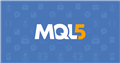# Mql5 price[ ] ?

Hey guys, I looked at the mql5 code of the rsi which I want to modify a bit. The OnCalculate function there have this in parameters const … price[] and inside the OnCalc function I see that array used but I don’t know exactly what it returns and want to know what it returns exactly. Sorry for not putting a code to explain I am typing on phone and can’t connect on computer for now.
Thanks for help. I want to know what that price array returns so I can create something to mimic it

And also how during the program functioning can I know which applied price was chosen. Is there a function that returns it?

Place the cursor on OnCalculaate() and press F1 - it'll tell you what you need.

Beside that here is the list of all function with short descriptions - for a keyword search.Documentation on MQL5: List of MQL5 Functions
• www.mql5.com
List of MQL5 Functions - MQL5 Reference - Reference on algorithmic/automated trading language for MetaTrader 5

When in doubt, always remember to reference the documentation. All of the following information is quoted from there.

OnCalculate returns ...

Return Value

int type value to be passed as the prev_calculated parameter during the next function call.

As for the price[] data, you will not be able to know what it is.

If you prefer to have access to the full OHLC data instead of a user selected price data, then use the other form of OnCalculate. There are two forms ...

# OnCalculate

The function is called in the indicators when the Calculate event occurs for processing price data changes. There are two function types. Only one of them can be used within a single indicator.

Calculation based on data array

 ```int  OnCalculate(    const int        rates_total,       // price[] array size    const int        prev_calculated,   // number of handled bars at the previous call    const int        begin,             // index number in the price[] array meaningful data starts from    const double&    price[]            // array of values for calculation    );```

Calculations based on the current timeframe timeseries

 ```int  OnCalculate(    const int        rates_total,       // size of input time series    const int        prev_calculated,   // number of handled bars at the previous call    const datetime&  time{},            // Time array    const double&    open[],            // Open array    const double&    high[],            // High array    const double&    low[],             // Low array    const double&    close[],           // Close array    const long&      tick_volume[],     // Tick Volume array    const long&      volume[],          // Real Volume array    const int&       spread[]           // Spread array    );```

Fernando Carreiro #:

When in doubt, always remember to reference the documentation. All of the following information is quoted from there.

OnCalculate returns ...

As for the price[] data, you will not be able to know what it is.

If you prefer to have access to the full OHLC data instead of a user selected price data, then use the other form of OnCalculate. There are two forms ...

Thanks
Reason: